Скачать презентацию Probability and Statistics Copyright Cengage Learning All

5073ff3a6c28a574789ed15c550fdd18.ppt

• Количество слайдов: 43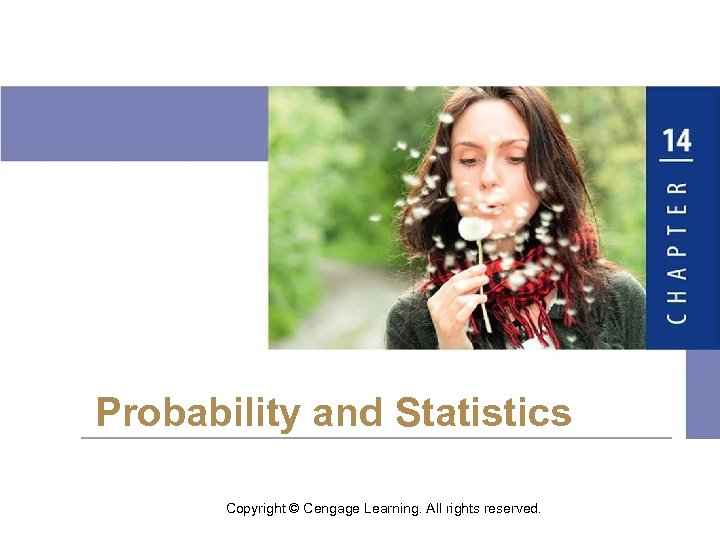Probability and Statistics Copyright © Cengage Learning. All rights reserved.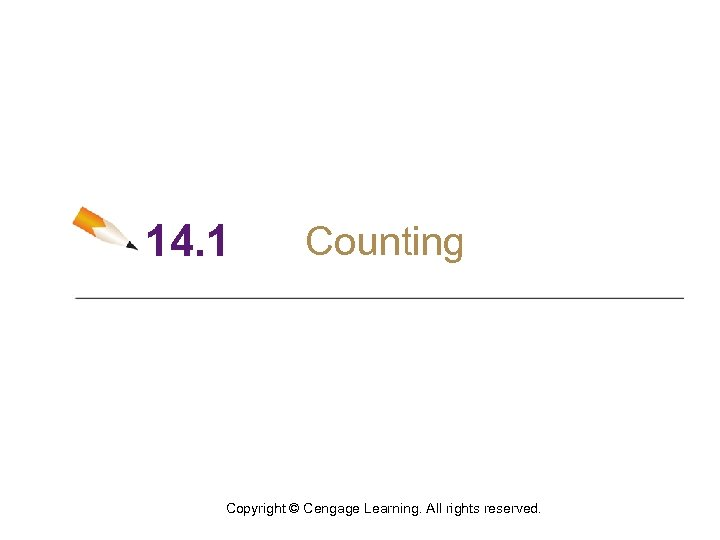14. 1 Counting Copyright © Cengage Learning. All rights reserved.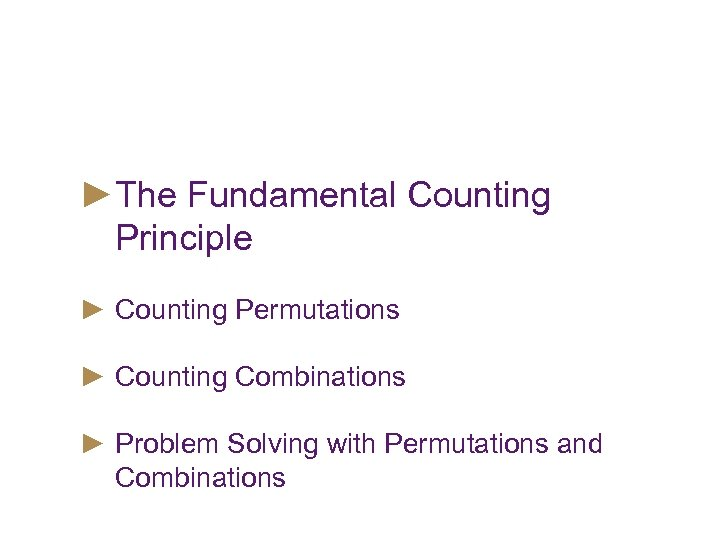Objectives ►The Fundamental Counting Principle ► Counting Permutations ► Counting Combinations ► Problem Solving with Permutations and Combinations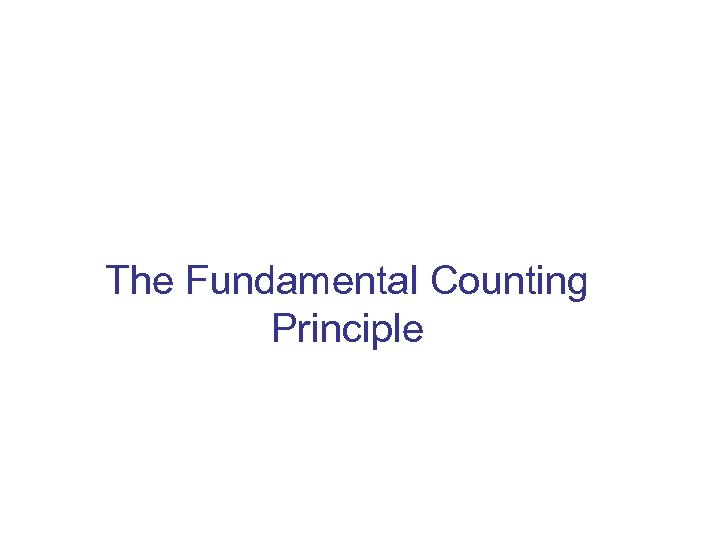The Fundamental Counting Principle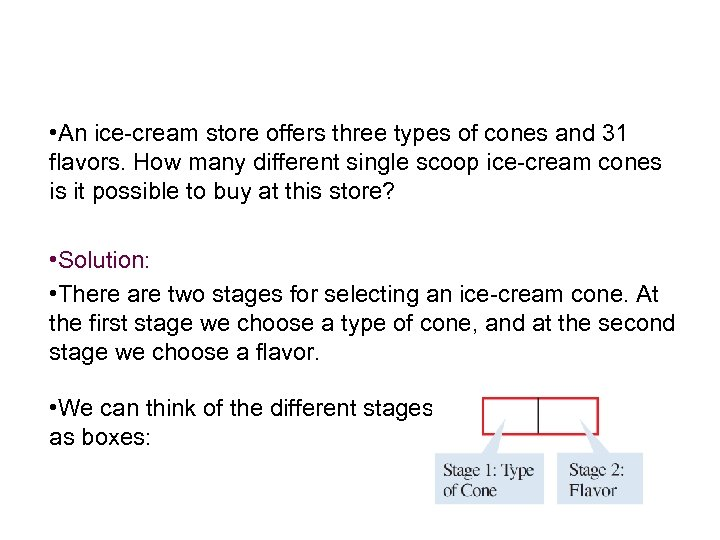Example 1 – Using the Fundamental Counting Principle • An ice-cream store offers three types of cones and 31 flavors. How many different single scoop ice-cream cones is it possible to buy at this store? • Solution: • There are two stages for selecting an ice-cream cone. At the first stage we choose a type of cone, and at the second stage we choose a flavor. • We can think of the different stages as boxes: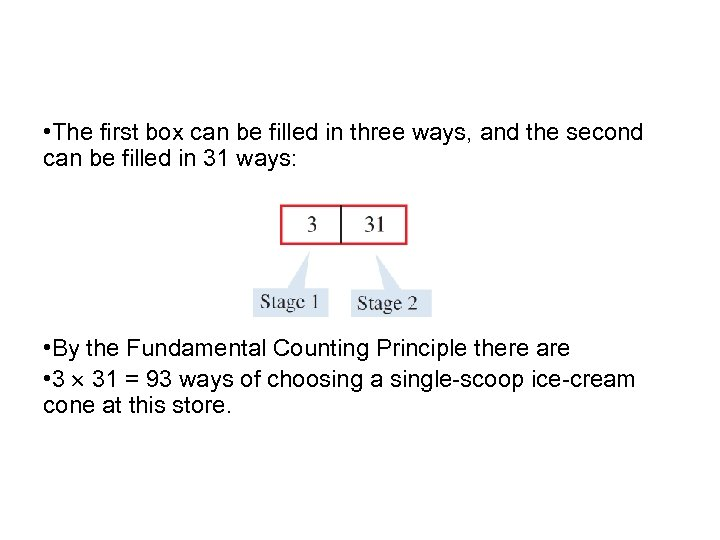Example 1 – Solution • The first box can be filled in three ways, and the second can be filled in 31 ways: • By the Fundamental Counting Principle there are • 3 31 = 93 ways of choosing a single-scoop ice-cream cone at this store. cont’dThe Fundamental Counting Principle • There is an immediate consequence of this principle for any number of events: If E 1, E 2, …. , Ek are events that occur in order and if E 1 can occur in n 1 ways, E 2 in n 2 ways, and so on, then the events can occur in order in n 1 n 2 …. nk ways.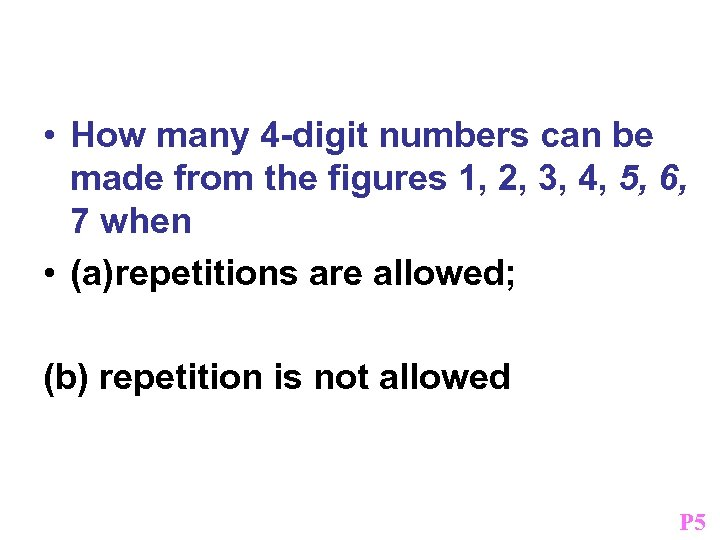Example • How many 4 -digit numbers can be made from the figures 1, 2, 3, 4, 5, 6, 7 when • (a)repetitions are allowed; (b) repetition is not allowed P 5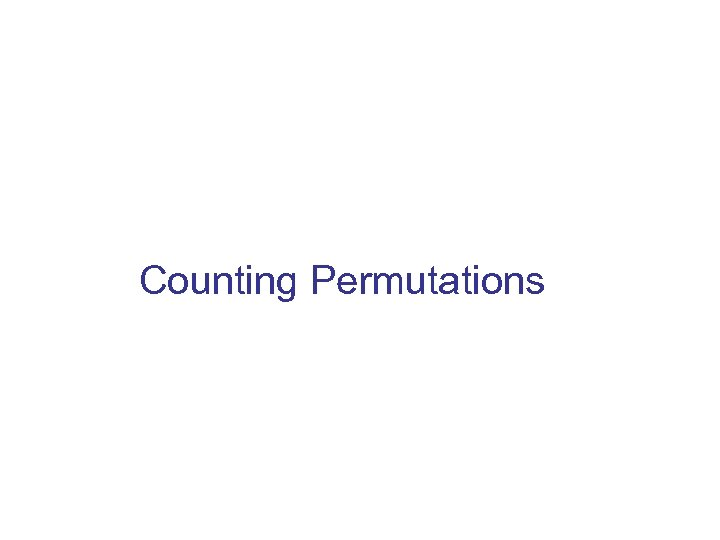Counting Permutations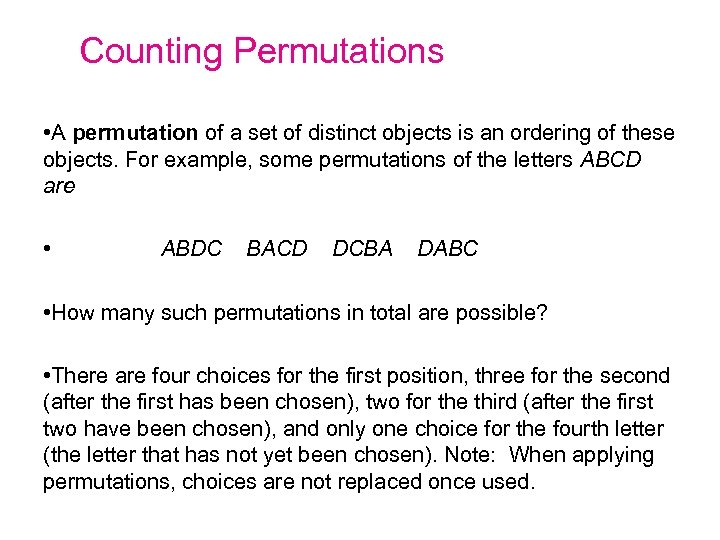Counting Permutations • A permutation of a set of distinct objects is an ordering of these objects. For example, some permutations of the letters ABCD are • ABDC BACD DCBA DABC • How many such permutations in total are possible? • There are four choices for the first position, three for the second (after the first has been chosen), two for the third (after the first two have been chosen), and only one choice for the fourth letter (the letter that has not yet been chosen). Note: When applying permutations, choices are not replaced once used.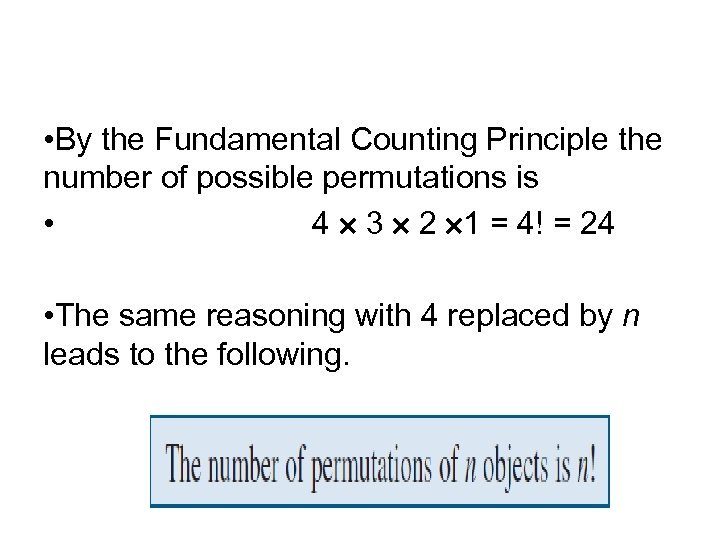Counting Permutations • By the Fundamental Counting Principle the number of possible permutations is • 4 3 2 1 = 4! = 24 • The same reasoning with 4 replaced by n leads to the following.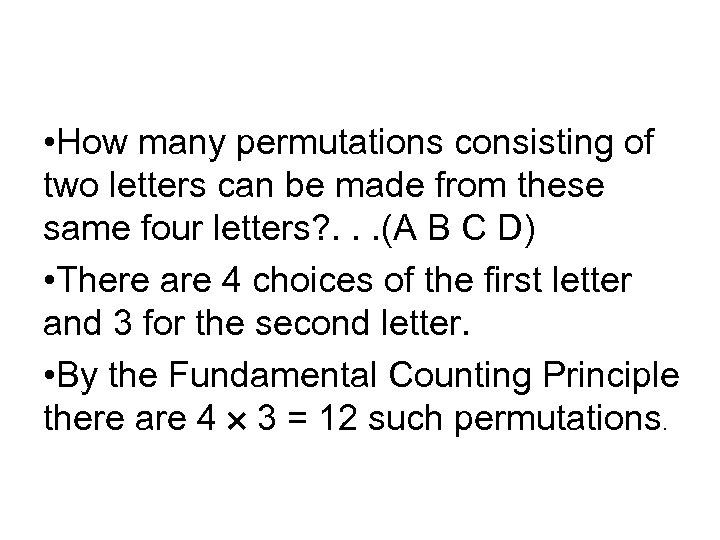Counting Permutations • How many permutations consisting of two letters can be made from these same four letters? . . . (A B C D) • There are 4 choices of the first letter and 3 for the second letter. • By the Fundamental Counting Principle there are 4 3 = 12 such permutations.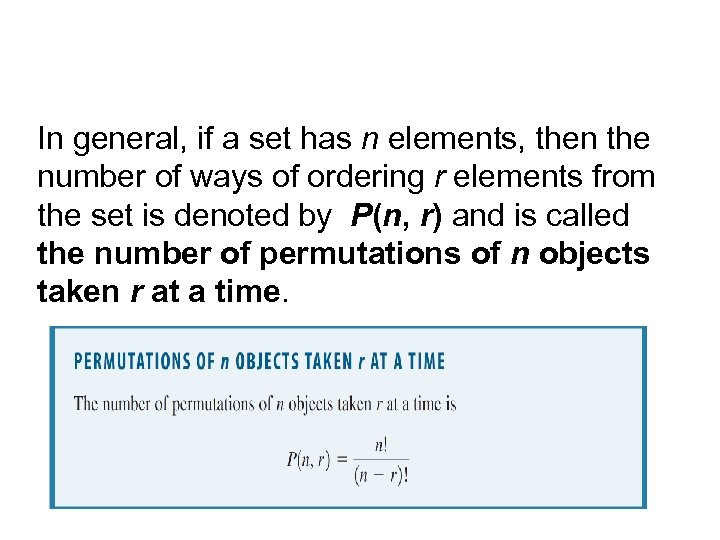In general, if a set has n elements, then the number of ways of ordering r elements from the set is denoted by P(n, r) and is called the number of permutations of n objects taken r at a time.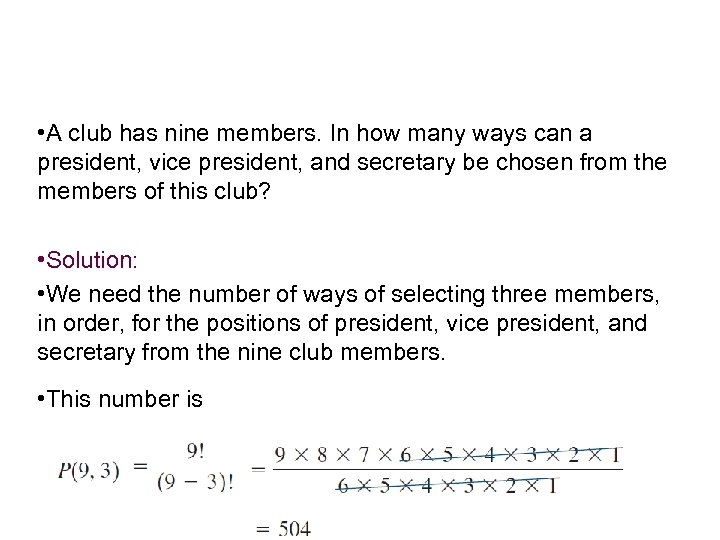Example 5 – Finding the Number of Permutations • A club has nine members. In how many ways can a president, vice president, and secretary be chosen from the members of this club? • Solution: • We need the number of ways of selecting three members, in order, for the positions of president, vice president, and secretary from the nine club members. • This number is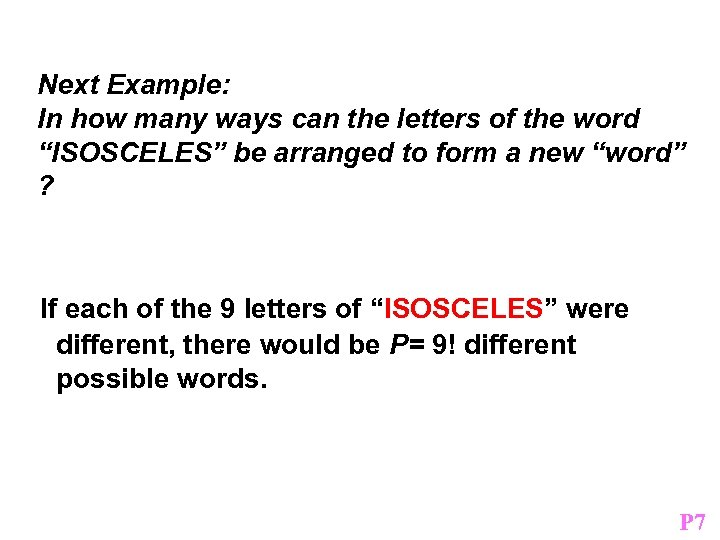Next Example: In how many ways can the letters of the word “ISOSCELES” be arranged to form a new “word” ? If each of the 9 letters of “ISOSCELES” were different, there would be P= 9! different possible words. P 7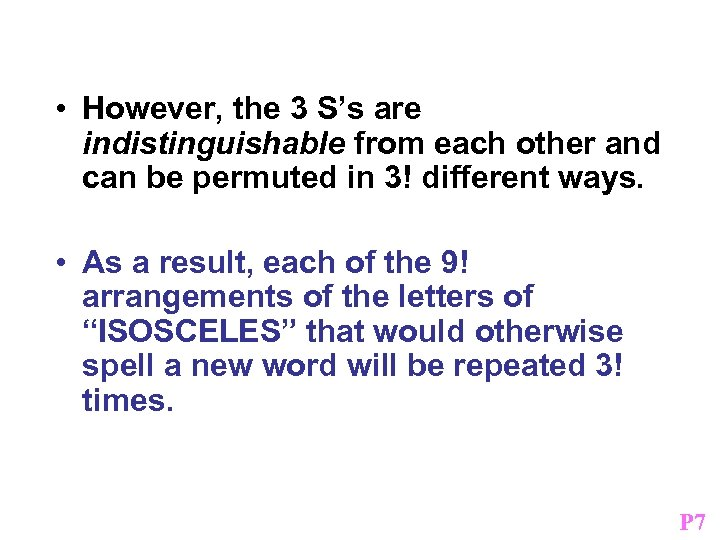• However, the 3 S’s are indistinguishable from each other and can be permuted in 3! different ways. • As a result, each of the 9! arrangements of the letters of “ISOSCELES” that would otherwise spell a new word will be repeated 3! times. P 7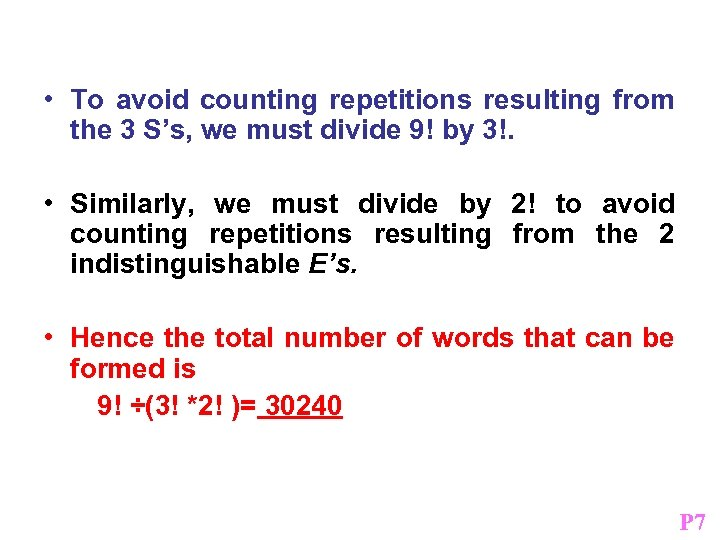• To avoid counting repetitions resulting from the 3 S’s, we must divide 9! by 3!. • Similarly, we must divide by 2! to avoid counting repetitions resulting from the 2 indistinguishable E’s. • Hence the total number of words that can be formed is 9! ÷(3! *2! )= 30240 P 7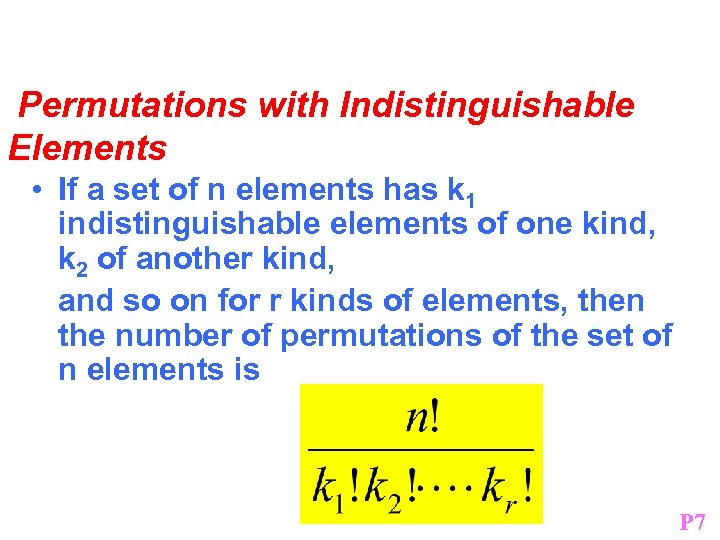Permutations with Indistinguishable Elements • If a set of n elements has k 1 indistinguishable elements of one kind, k 2 of another kind, and so on for r kinds of elements, then the number of permutations of the set of n elements is P 7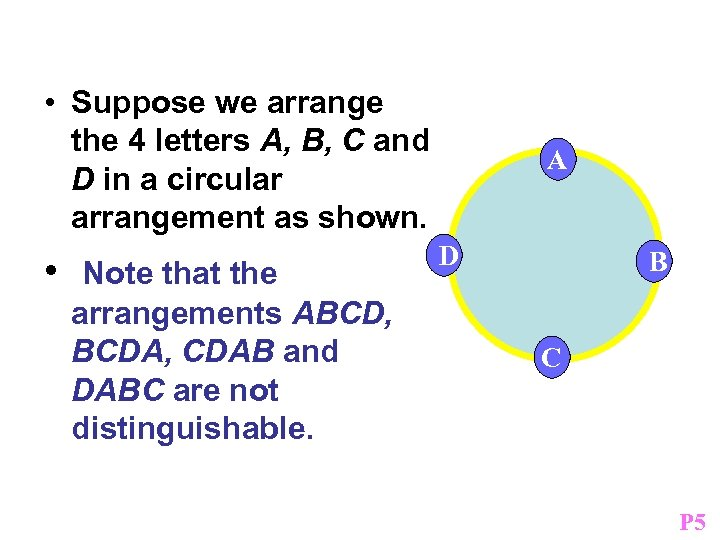• Suppose we arrange the 4 letters A, B, C and D in a circular arrangement as shown. • Note that the arrangements ABCD, BCDA, CDAB and DABC are not distinguishable. A D B C P 5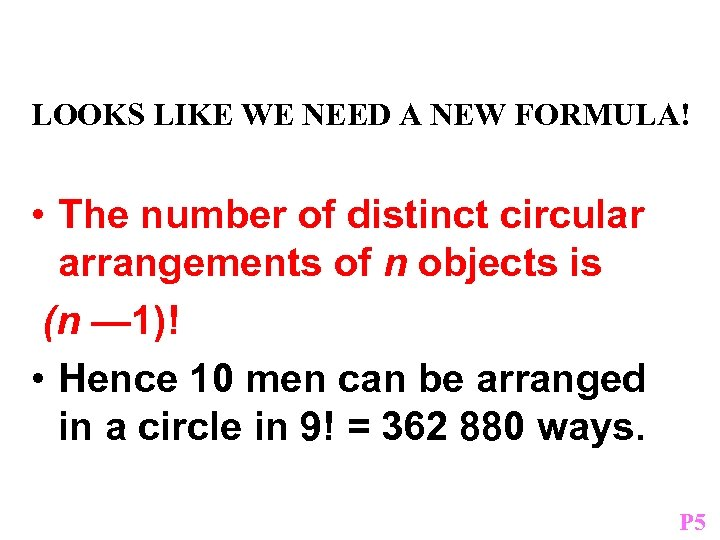LOOKS LIKE WE NEED A NEW FORMULA! • The number of distinct circular arrangements of n objects is (n — 1)! • Hence 10 men can be arranged in a circle in 9! = 362 880 ways. P 5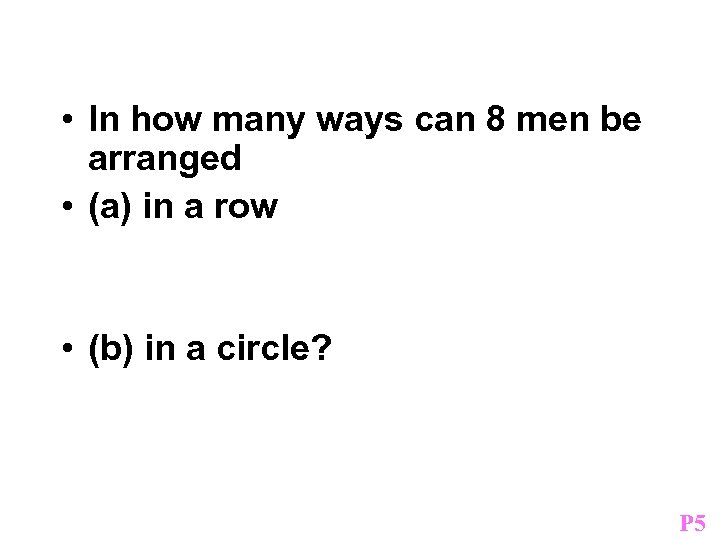Example • In how many ways can 8 men be arranged • (a) in a row • (b) in a circle? P 5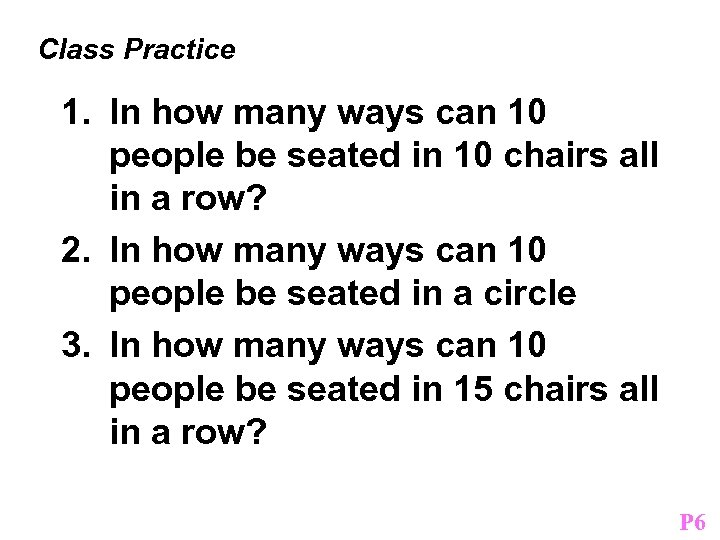Class Practice 1. In how many ways can 10 people be seated in 10 chairs all in a row? 2. In how many ways can 10 people be seated in a circle 3. In how many ways can 10 people be seated in 15 chairs all in a row? P 6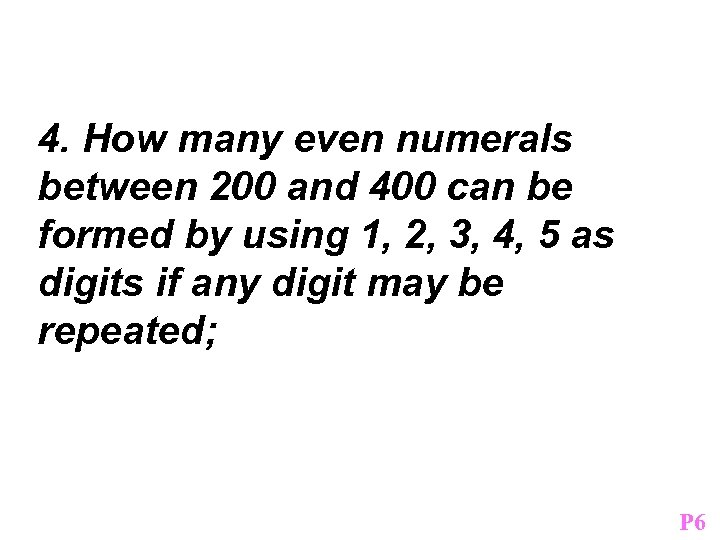4. How many even numerals between 200 and 400 can be formed by using 1, 2, 3, 4, 5 as digits if any digit may be repeated; P 6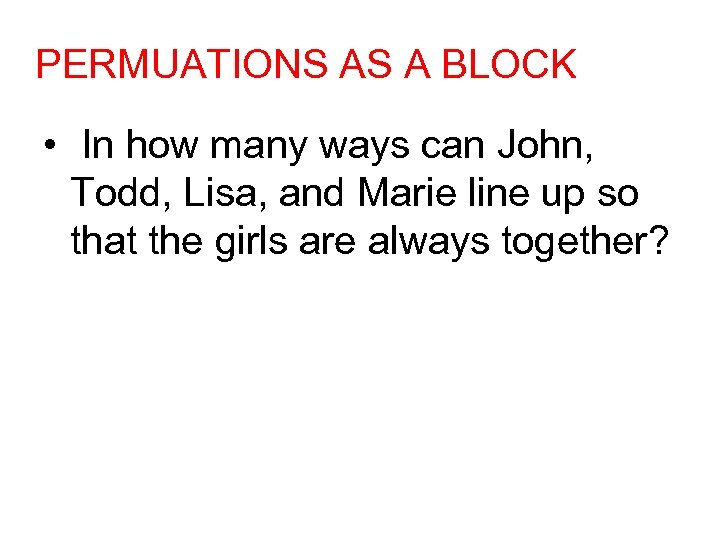PERMUATIONS AS A BLOCK • In how many ways can John, Todd, Lisa, and Marie line up so that the girls are always together?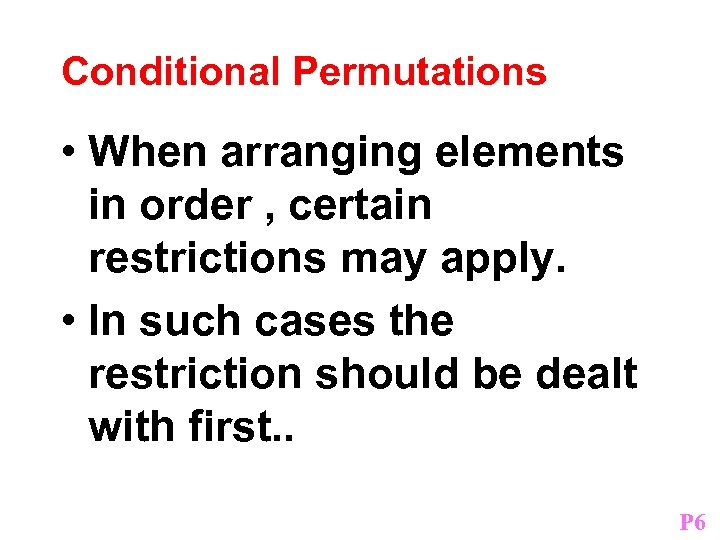Conditional Permutations • When arranging elements in order , certain restrictions may apply. • In such cases the restriction should be dealt with first. . P 6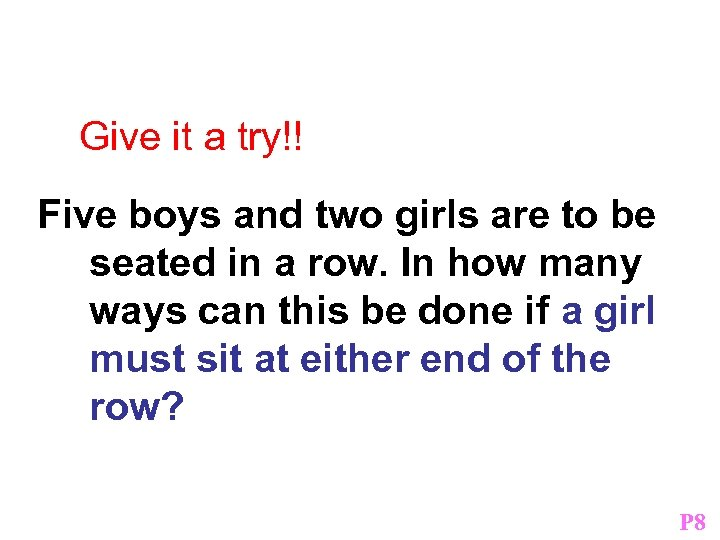Ces Practice Give it a try!! Five boys and two girls are to be seated in a row. In how many ways can this be done if a girl must sit at either end of the row? P 8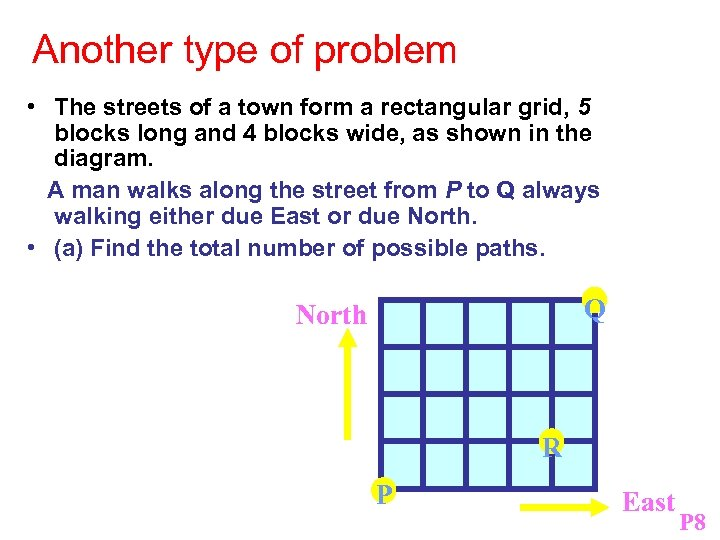Another type of problem! • The streets of a town form a rectangular grid, 5 blocks long and 4 blocks wide, as shown in the diagram. A man walks along the street from P to Q always walking either due East or due North. • (a) Find the total number of possible paths. Q North R P East P 8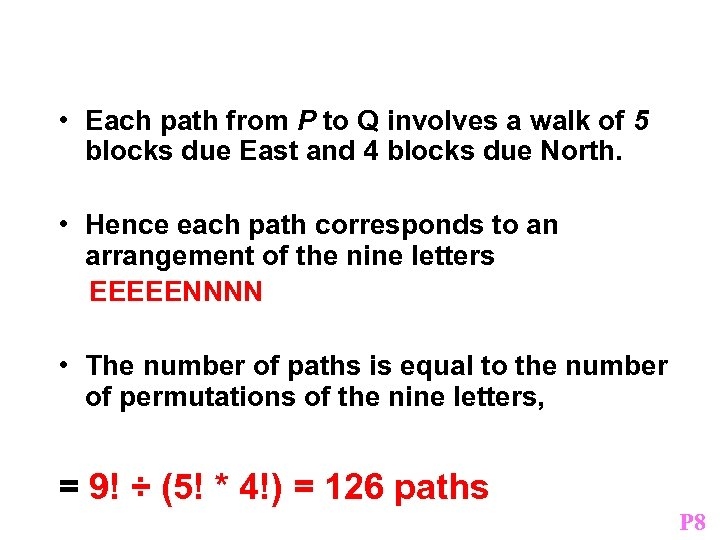• Each path from P to Q involves a walk of 5 blocks due East and 4 blocks due North. • Hence each path corresponds to an arrangement of the nine letters EEEEENNNN • The number of paths is equal to the number of permutations of the nine letters, = 9! ÷ (5! * 4!) = 126 paths P 8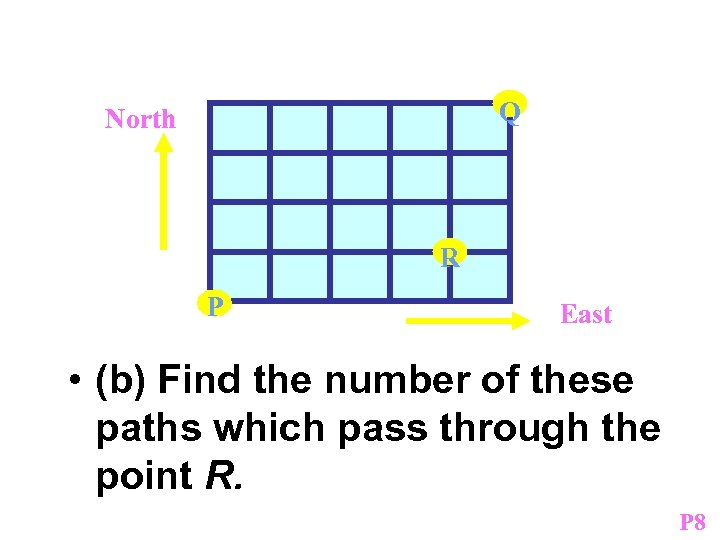Q North R P East • (b) Find the number of these paths which pass through the point R. P 8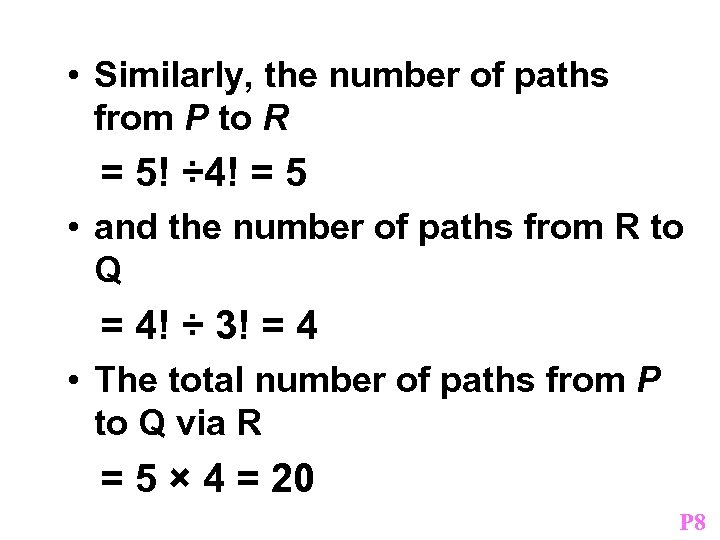• Similarly, the number of paths from P to R = 5! ÷ 4! = 5 • and the number of paths from R to Q = 4! ÷ 3! = 4 • The total number of paths from P to Q via R = 5 × 4 = 20 P 8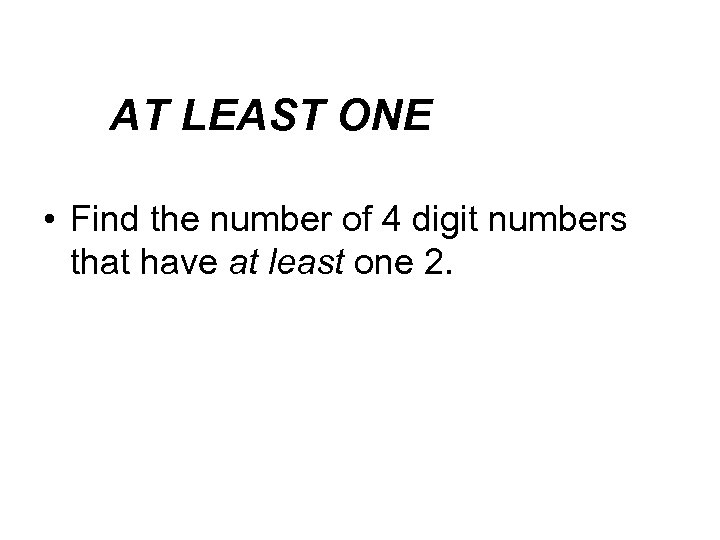AT problems with Solving LEAST ONE “ AT LEAST” • Find the number of 4 digit numbers that have at least one 2.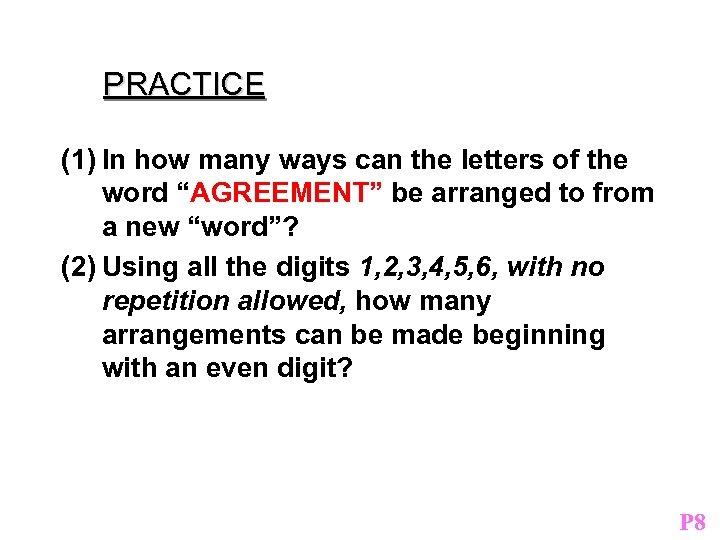PRACTICE (1) In how many ways can the letters of the word “AGREEMENT” be arranged to from a new “word”? (2) Using all the digits 1, 2, 3, 4, 5, 6, with no repetition allowed, how many arrangements can be made beginning with an even digit? P 8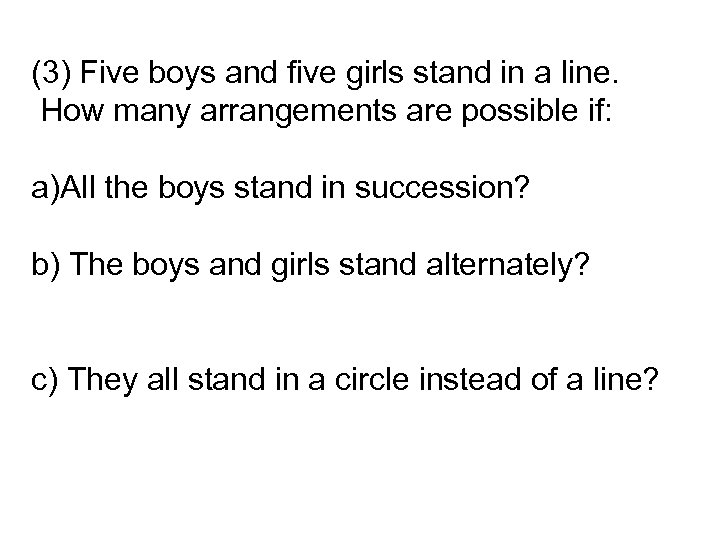(3) Five boys and five girls stand in a line. How many arrangements are possible if: a)All the boys stand in succession? b) The boys and girls stand alternately? c) They all stand in a circle instead of a line?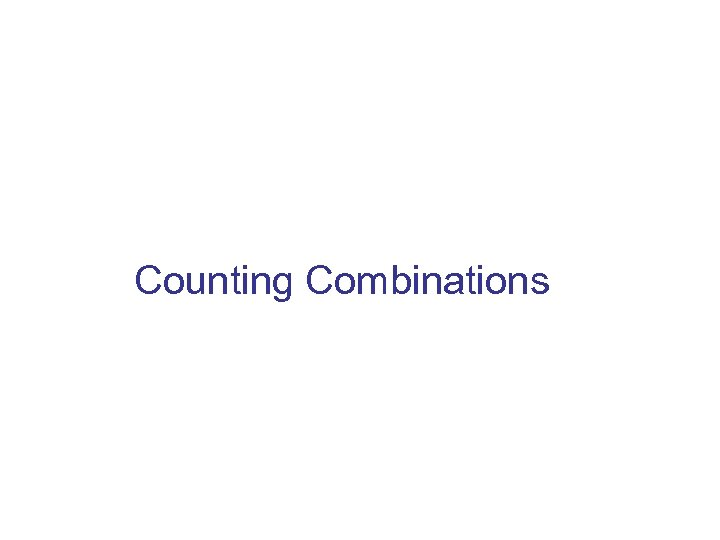Counting Combinations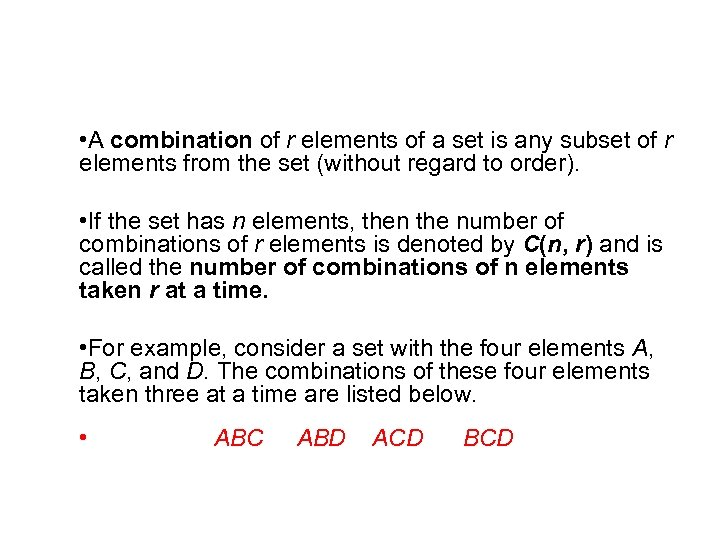Counting Combinations • A combination of r elements of a set is any subset of r elements from the set (without regard to order). • If the set has n elements, then the number of combinations of r elements is denoted by C(n, r) and is called the number of combinations of n elements taken r at a time. • For example, consider a set with the four elements A, B, C, and D. The combinations of these four elements taken three at a time are listed below. • ABC ABD ACD BCD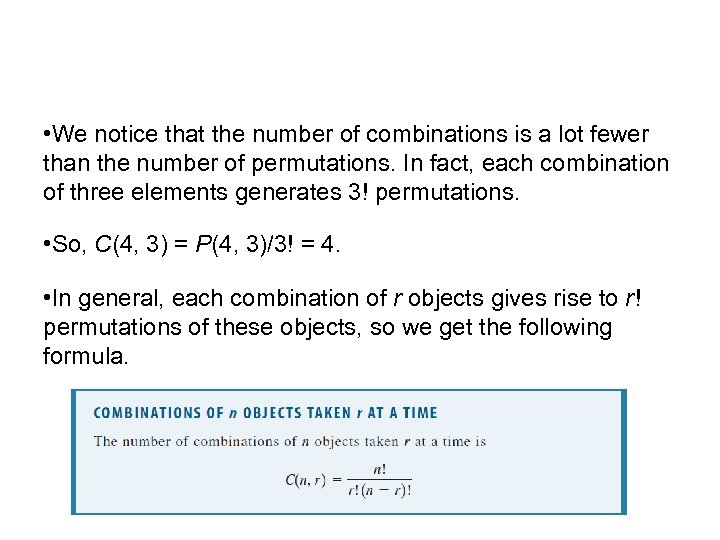Counting Combinations • We notice that the number of combinations is a lot fewer than the number of permutations. In fact, each combination of three elements generates 3! permutations. • So, C(4, 3) = P(4, 3)/3! = 4. • In general, each combination of r objects gives rise to r ! permutations of these objects, so we get the following formula.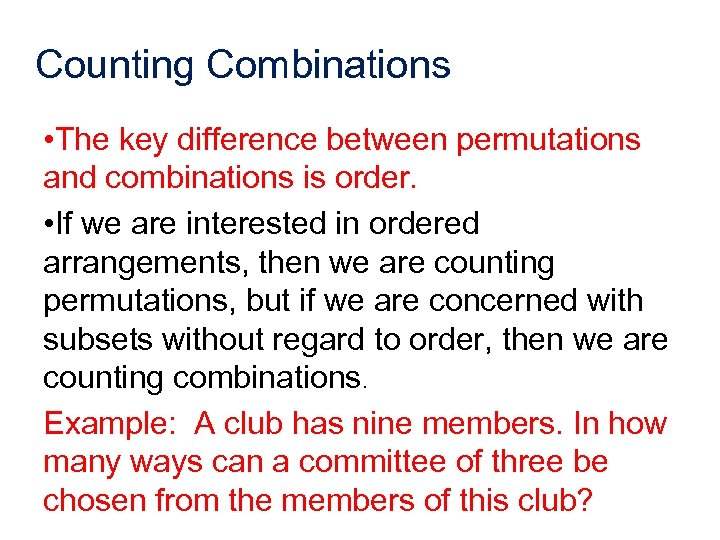Counting Combinations • The key difference between permutations and combinations is order. • If we are interested in ordered arrangements, then we are counting permutations, but if we are concerned with subsets without regard to order, then we are counting combinations. Example: A club has nine members. In how many ways can a committee of three be chosen from the members of this club?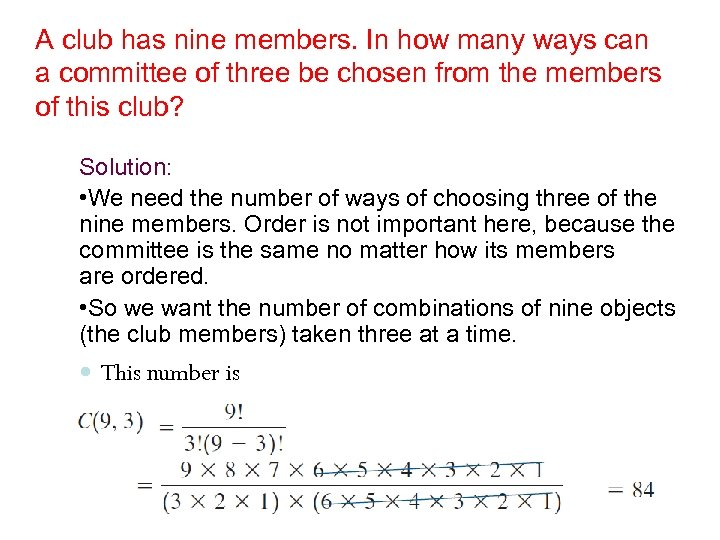A club has nine members. In how many ways can a committee of three be chosen from the members of this club? Solution: • We need the number of ways of choosing three of the nine members. Order is not important here, because the committee is the same no matter how its members are ordered. • So we want the number of combinations of nine objects (the club members) taken three at a time. This number is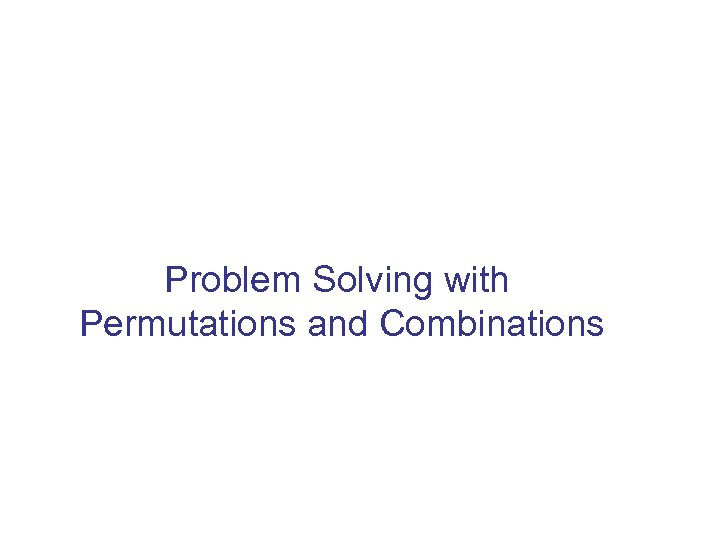Problem Solving with Permutations and Combinations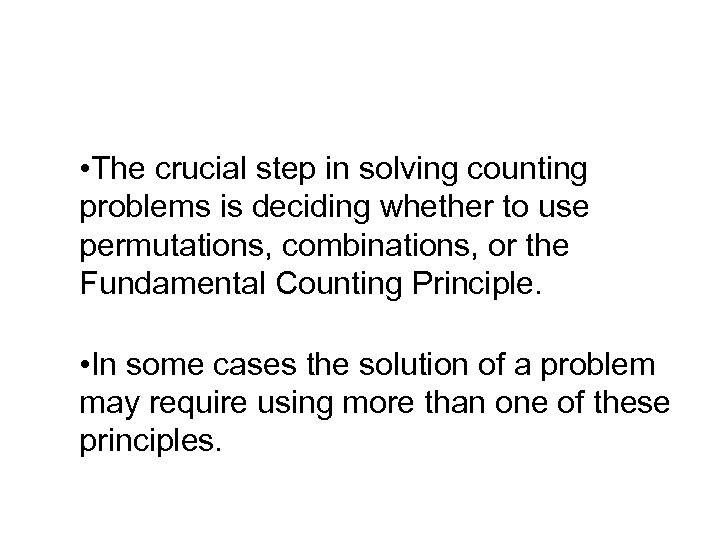Problem Solving with Permutations and Combinations • The crucial step in solving counting problems is deciding whether to use permutations, combinations, or the Fundamental Counting Principle. • In some cases the solution of a problem may require using more than one of these principles.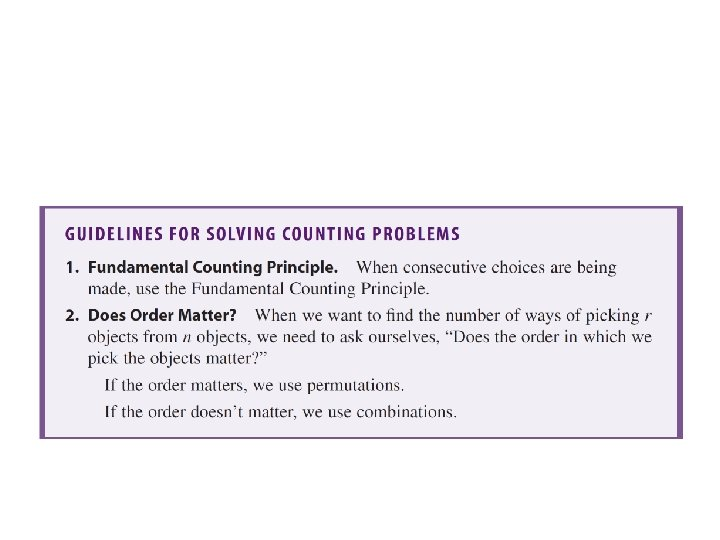Problem. Here are some general guidelines to help us decide how to apply these principles. Solving with Permutations and Combinations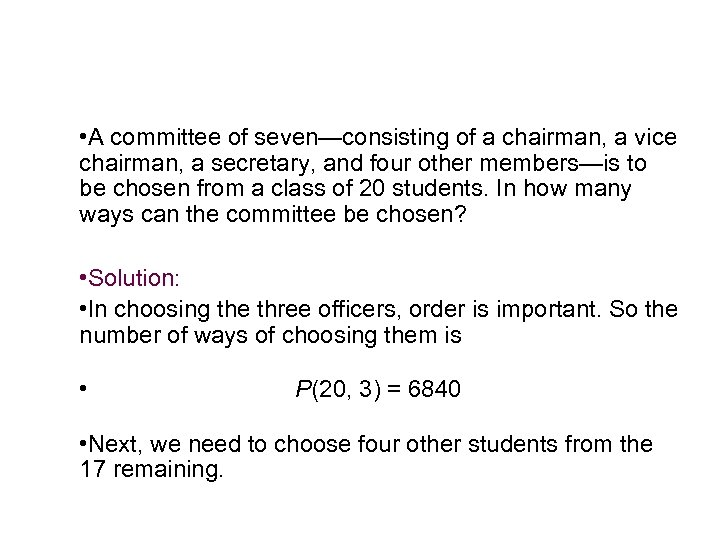Example 10 – Using Permutations and Combinations • A committee of seven—consisting of a chairman, a vice chairman, a secretary, and four other members—is to be chosen from a class of 20 students. In how many ways can the committee be chosen? • Solution: • In choosing the three officers, order is important. So the number of ways of choosing them is • P(20, 3) = 6840 • Next, we need to choose four other students from the 17 remaining.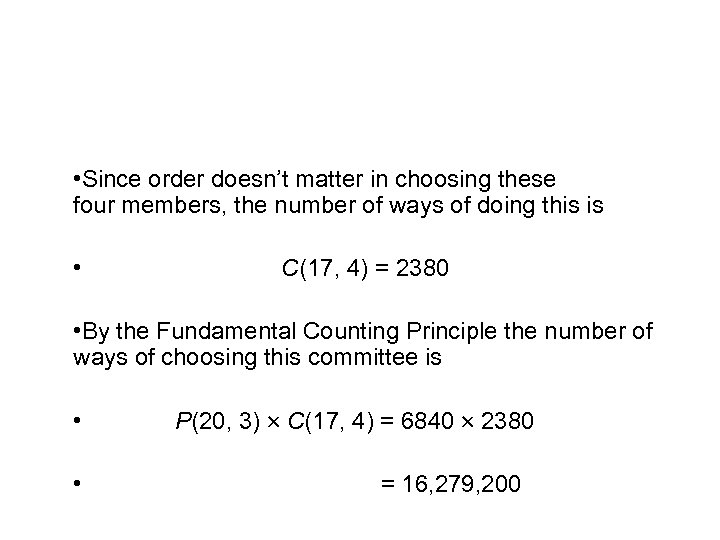cont’d Example 10 – Solution • Since order doesn’t matter in choosing these four members, the number of ways of doing this is • C(17, 4) = 2380 • By the Fundamental Counting Principle the number of ways of choosing this committee is • • P(20, 3) C(17, 4) = 6840 2380 = 16, 279, 200